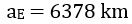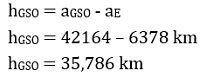# Geostationary Orbit

Geostationary Orbit refers to a circular orbit present above the earth’s equatorial plane possessing a period of revolution equivalent to the period of rotation of the earth. Here the term ‘geostationary’ corresponds to the orbit where the satellite appears to remain fixed at a point or stationary with respect to the surface of the earth.

It is to be kept in mind that here you must not get confused between satellite appearing stationary and in actuality being stationary. This is so because, in a geostationary orbit, the satellite moves but while in motion its speed is equal to the rotational speed of the earth, and hence from a specific point on the surface of the earth it is seen that the satellite is not moving.

Geostationary orbit is regarded as a special case of geosynchronous orbit which corresponds to an orbit whose orbital period is equivalent to earth’s rotation period.

## Content: Geostationary Orbit

### Introduction to Orbit

The idea of geostationary orbit was announced by a scientist cum author of British origin named Arthur C. Clarke in October 1945. Another name for this is Clarke Orbit.

In our previous content, satellite orbits we have defined that an orbit is a path around the earth over which the satellite is rotated around the earth. We have also discussed that if the satellite rotates in the same direction wrt the rotation of earth then it is known as prograde while if the direction of rotation of the satellite is opposite to the earth then it is called retrograde.

Basically, the motion of the satellite in the respective orbit is because of adequate balancing maintained between the centripetal force of the satellite and the force of gravity. However, this balancing depends on factors like the speed with which the satellite is moving, the gravitational pull and radius of the earth, and the distance from the surface of the earth.

The radius of the earth corresponds to the distance between the center of the earth and a satellite orbiting in space whereas the altitude denotes the height of separation from satellite to the earth surface. The inclination corresponds to the rotation angle of the satellite in correspondence with the horizon.

A major classification of orbits is done as:

• GEO
• MEO
• LEO

In this content, we will have a detailed idea of geostationary orbit.

## What is Geostationary Orbit?

At the beginning itself, we have discussed that a geostationary orbit is the one where the satellite appears to be immobile to the earth-based observer. However, recently we have seen that satellites can move in three different orbits around the earth.

There are three requirements of an orbit to be geostationary. These are as follows:

1. The satellite must have a rotational speed similar to that of earth, traveling in an eastward direction.
2. The shape of the orbit must be circular.
3. The orbit must possess zero inclination.

Let us now understand each condition separately:

The first discussed condition is the base of this orbital system due to the fact that in order to appear stationary with respect to the earth, it is necessary that the satellite must travel with the rotational speed of the earth. So, the satellites moving in the geostationary orbit circle the complete earth in around 23 hours 56 minutes, and 4 seconds. This corresponds that the satellite is moving at the same speed with which the earth is rotating. Due to this reason, the satellite is seen as immobile.

The second listed condition is a circular orbit, and it is somewhat can be understood from the first condition and Kepler’s second law. As we have discussed in the first condition that the speed of the satellite will remain constant and an invariable speed shows that the satellites moving with constant speed will cover equal areas in equal time duration and this is achievable in the case of circular orbits only.According to the last condition, the inclination must be zero. If there will be any inclination, then the motion of the satellite will be north and south, thus the orbit will not be geostationary. The north and south movement can be eliminated with zero inclination showcasing that the orbit is present in the equatorial plane of earth.

### Geostationary Satellite Height

While discussing Kepler’s three laws, we have discussed in the third law that the square of the periodic time of the satellite in the orbit is proportional to the cube of the semi-major axis i.e., ‘a’ for a circular orbit. This means that the orbital period of the satellite increases with the increase in the radius of the orbit. On representing the radius of geostationary orbit as aGSO, we can have,: P represents the period of geostationary orbit i.e., 23 hr, 56 min, and 4 s, which means the solar time. This is nothing other than the time which is taken by the earth to complete one revolution about its N-S axis,

μ denotes the earth’s geocentric gravitational constant having value 3.98 * 1014 m3/s2.

So, on substituting the values of P and μ in the above equation, we will have,While the equatorial radius of the earth is nearly,Thus, the height of the geostationary orbit in consideration with the surface of the earth will be,Hence, we can say that the geostationary orbit is present at a height of about 36,000 km from the earth’s equator.

• Antenna tracking or re-orientation of the antenna on a ground station is not required for the satellite in geostationary orbit as here the satellite appears to be of immobile nature. Thus, the accurate pointing of the antenna beam can be achieved by using elevation and azimuth angles.
• At such a great height, large coverage area-wise is achieved. Also, an elevation angle of 5⁰ provides 38% of the coverage area.
• A total of three geostationary satellites at an angle of separation of 120⁰ can cover the whole surface area of the earth.